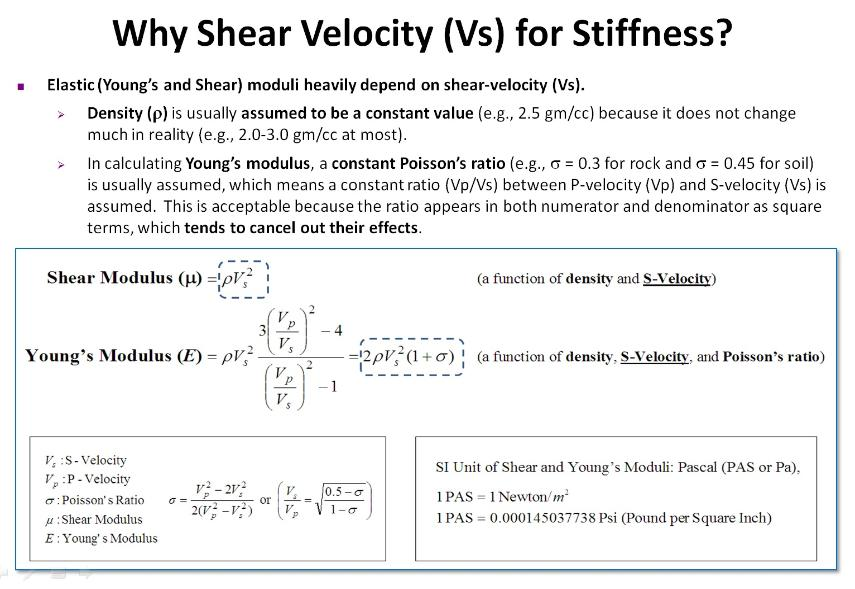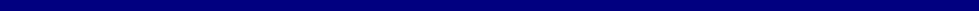e-mail: contact@masw.com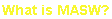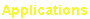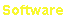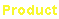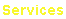Shear-Wave Velocity (Vs) for Stiffness of Material

Most geotechnical engineering projects deal with material's stiffness.  Both conventional and modern approaches are currently being used for this
purpose, such as standard penetration test (SPT) and seismic cone penetration testing (SCPT).  They are empirical methods.  Scientifically,
stiffness of a material is defined as a measure of resistance to deformation (
Sheriff, 2002) and ultimately related to material's elastic moduli that
describe the material's behavior under stress.  Among the three primary types of moduli—Young's (E), shear (µ), and bulk (B)—the first two (E and
µ) are most commonly used because of what they represent.  Young's modulus (E) simply dictates the deformation resistance along the axis of
stress, whereas the shear modulus (µ) indicates the resistance to shape deformation (i.e., shearing) that, in turn, is related to the viscosity
property.  The bulk modulus (B) is related to the resistance to volume change.  In reality, deformation always accompanies both transverse and
longitudinal changes only at a different ratio, and the volume change can be vastly ignored in geotechnical engineering.  In this sense, the most
comprehensive and accurate definition of stiffness should include both moduli of E and µ.  According to the theory of elasticity (
Sheriff and Geldart,
1982), these two moduli can be defined by a material's density and the two seismic velocities (or by Poisson's ratio) of Vp (P-wave) and Vs (S-
wave).  Defining equations indicate the heaviest dependency of both moduli on Vs as illustrated in the figure below.  This is why seismic shear-wave
velocity (Vs) is often used as a direct indicator of a material's stiffness.

Accurate measurement of seismic velocities (Vp and Vs) has been one of the most important subjects in the history of theoretical and exploration
geophysics.  A variety of methods have been used for this purpose, such as seismic down-hole and cross-hole measurements and seismic
refraction and reflection surveys, with different degree of accuracy in results and also different costs in measurement and data analysis (
Dobrin,
1976).  However, it is a common obstacle for all of these methods to struggle against the low signal-to-noise ratio (SN) in general, which is inevitable
in all types of seismic measurements employing body (P and S) waves.  Recent introduction of the multichannel analysis of surface waves (MASW)
method (
Park et al., 1999) has made it possible to make a highly accurate evaluation of shear-wave velocity (Vs) at only a fraction of the cost of
what is needed for conventional body-wave methods.  This is possible because MASW utilizes seismic surface waves that always maintain an
energy level higher than that of body waves by a few orders of magnitude.  It has been rapidly adopted for various types of unique and challenging
projects within the geotechnical engineering community since its first introduction in the late 1990s.